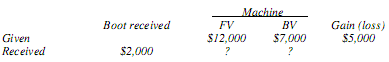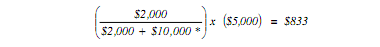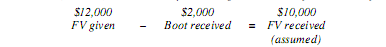Connect with us

# Non-monetary Exchanges of Fixed AssetPublished

onNonmonetary transactions covered under APB 29 and FASB Statement No. 153 deal primarily with exchanges or distributions of fixed assets. Fair market value in a nonmonetary exchange may be based on:  Quoted market price, Appraisal, Cash transaction for similar items. As per FASB Statement No. 153 (Exchanges of Non-monetary Assets), the asset received in a nonmonetary exchange is recorded at fair market value when the transaction has commercial substance. Commercial substance exists when future cash flows change because of the transaction arising from a change in economic positions of the two parties. A gain or loss is recorded for the difference between the book value of the asset given up and the fair market value of the asset received. However, if commercial substance does not exist, the exchange is recorded based on book values with no gain or loss recognized.

It sounds simple, doesn’t it? How if cash or boot or both of them is involved in the exchange transaction? Not simple anymore. Through this post I try to discuss this issue with some case examples in various scenarios to be applicable to various possibilities of asset exchanges. Enjoy!

The exchange of nonmonetary assets (such as inventory, property, and equipment) for other non-monetary assets requires special consideration of two amounts:

.  Gain or loss, if any
.  Fair market value of the nonmonetary assets received

Gain or loss on a nonmonetary exchange is computed as follows:

Fair value of the asset given (FV) – Book value of the asset given (BV) = Gain (loss)

If the fair value (FV) of the asset given up cannot be determined, assume it is equal to the fair value of the asset received.  If neither fair values are determinable, gain or loss cannot be computed. The asset received is generally recorded at the fair value of the asset surrendered [or the FV of the asset received if “more clearly evident”].  Some exceptions do exist.  Remember, however, that the asset given up will always be removed from the books at book value.

The following rules apply in recording nonmonetary exchanges:

• Losses are always recognized (conservatism).
• Gains are recognized when dissimilar assets are exchanged (i.e., a machine for a truck).
• Gains are not recognized when similar assets are exchanged (i.e., a machine for a machine) because the earnings process is not considered complete.
• The asset received is recorded at the fair value of the asset given up (or the FV of the asset received if “more clearly evident”) whenever gains and losses are recognized.
• The asset received is recorded at the book value of the asset given up when gains and losses are not recognized.  Gains and losses are not recognized when (1) the earnings process is not considered complete, and (2) gain or loss cannot be computed.

### When Boot or Cash Involved in Exchange of Fixed Asset

Exceptions to these rules occur when boot (cash) is involved in the exchange. Guys, please bear with me. Read on…

### Scenario 1. Fair Value Is Not Determinable

A company trades a machine (cost, \$10,000; accumulated depreciation, \$3,000) for land. Neither the FV of the machine or the land is determinable. The journal entry is:

[Debit]. Land = 7,000  (BV given)
[Debit]. Accumulated depreciation = 3,000
[Credit]. Machine = 10,000
Note: BV = Book Value

Since FV is unknown, no gain can be computed, and the asset received is recorded at the BV of the asset given up (\$10,000 – \$3,000).

### Scenario 2. Loss, No Boot Involved

EXAMPLE:  A company trades a machine with a FV of \$5,000 (cost, \$10,000; accumulated depreciation, \$3,000) for land.
FV         BV           Gain (loss)
Machine given    \$5,000  \$7,000     \$(2,000)

The loss (FV – BV) is recognized immediately and the land is recorded at the FV of the machine given up. And the journal entry is:

[Debit]. Land = 5,000 (FV given)
[Debit]. Accumulated depreciation = 3,000
[Debit]. Loss = 2,000
[Credit]. Machine  = 10,000
(Note that this transaction would be treated the same way if similar assets had been exchanged).

Anoher variation of this scenario:  A company trades a machine (cost, \$10,000; accumulated depreciation, \$3,000) for another machine with a FV of \$6,000.
FV        BV             Gain (loss)
Machine given                            \$7,000     \$(1,000)

In this case, the FV of the asset given is unknown.  You can substitute the FV of the asset received for FV given when computing gain/loss.  The fair value of the asset given is implied in this case.

\$6,000         \$7,000        \$(1,000)
FV given  –  BV given  =  Loss
[assumed or implied]

The journal entry is:

[Debit]. Machine = 6,000  (FV received)
[Debit]. Accumulated depreciation = 3,000
[Debit]. Loss = 1,000
[Credit]. Machine  = 10,000

### Scenario 3. Loss, Boot Given

A company trades a machine with a FV of \$6,000 (cost, \$10,000; accumulated depreciation, \$3,000) and \$500 cash for land.
Boot     FV          BV          Gain (loss)
Machine given          \$500    \$6,000   \$7,000   \$(1,000)

Gain (loss) still equals FV less BV.  Boot given is added to the value of the asset given. The journal entry is:

[Debit]. Land  = 6,500    (FV given + Boot given)
[Debit]. Accumulated depreciation = 3,000
[Debit]. Loss = 1,000
[Credit]. Machine  = 10,000
[Credit]. Cash  = 500

Let’s explore further by assuming the FV of the machine was unknown, but the FV of the land received was known to be \$6,000.
Boot         FV            BV                 Gain (loss)
Machine given        \$500                        \$7,000           \$(1,500)

To compute gain (loss), you can assume FV given is equal to FV received less boot given (\$6,000 – \$500).

\$5,500            \$7,000      \$(1,500)
FV given  –  BV given  =  Loss
(assumed)

And, the journal entry is:

[Debit]. Land = 6,000  (FV received)
[Debit]. Accumulated depreciation =  3,000
[Debit]. Loss = 1,500
[Credit]. Machine = 10,000
[Credit]. Cash = 500

### Scenario 4.  Loss, Boot Received

A company trades a machine with a FV of \$6,000 (cost, \$10,000; accumulated depreciation, \$3,000) for land and \$500 cash.
Boot            FV          BV         Gain (loss)
Machine given                           \$6,000   \$7,000   \$(1,000)

Boot received is deducted from the value of the asset given. And the journal entry is:

[Debit]. Land =  5,500    (FV given – Boot received)
[Debit]. Accumulated depreciation = 3,000
[Debit]. Cash = 500
[Debit]. Loss = 1,000
[Credit]. Machine = 10,000

Now let’s assume the FV of the machine is unknown but the FV of the land received is known to be \$6,000.
Boot        FV             BV           Gain (loss)
Machine given                                         \$7,000    \$(500)

In this case, you can assume FV given is equal to FV received plus the boot received (\$6,000 + \$500).

\$6,500          \$7,000         \$(500)
FV given  –  BV given  =  Loss
(assumed)

And the journal entry is:

[Debit]. Land = 6,000  (FV received)
[Debit]. Accumulated depreciation = 3,000
[Debit]. Cash = 500
[Debit]. Loss = 500
[Credit]. Machine = 10,000

### Scenario 5.  Gain, Dissimilar Assets

A company trades a computer with a FV of \$12,000 (cost, \$11,000; accumulated depreciation, \$4,000) for a building.
FV             BV          Gain (loss)
Computer given           \$12,000    \$7,000   \$5,000

Since the assets traded are dissimilar, the earnings process is considered complete and the entire gain is recognized. And the journal entry is:

[Debit]. Building = 12,000  (FV received)
[Debit]. Accumulated depreciation = 4,000
[Credit]. Computer = 11,000
[Credit]. Gain =  5,000

If boot had been involved, the accounting would be the same as that illustrated in the loss situation.

### Scenario 6. Gain, Similar Assets, No Boot Involved

A company trades equipment with a FV of \$12,000 (cost, \$11,000; accumulated depreciation, \$4,000) for similar equipment.
FV              BV          Gain (loss)
Equipment given                   \$12,000    \$7,000   \$5,000

Since similar assets have been traded, the earnings process is not considered complete and no gain is recognized.  The asset received is debited at the book value (not fair value) of the asset given. The journal entry is:

[Debit]. Equipment = 7,000 (BV given)
[Debit]. Accumulated depreciation = 4,000
[Credit]. Equipment  = 11,000

The unrecognized gain of \$5,000 will be deferred; it will be recognized as the equipment received is depreciated (lower depreciation results than if FV had been used) and/or sold or disposed of in a subsequent transaction.

### Scenario 7. Gain, Similar Assets, Boot Given

A company trades a machine (cost, \$10,000; accumulated depreciation, \$2,000) and \$4,000 in cash for a similar machine with a FV of \$16,000.
Boot          FV               BV             Gain (loss)
Machine given                \$4,000                         \$8,000      \$4,000

As in the loss situation, the FV of the asset given can be assumed equal to the FV received less the boot given (\$16,000 – \$4,000).

\$12,000       \$8,000        \$4,000
FV given  –  BV given  =  Gain
(assumed)

Boot given is added to the value of the asset received (in this case, book value).  Gain is still unrecognized. The journal entry is:

[Debit]. Machine  = 12,000  (BV given + Boot given)
[Debit]. Accumulated depreciation = 2,000
[Credit]. Machine  = 10,000
[Credit]. Cash  = 4,000

Below are alternative ways to calculate the asset received:

FV received        \$16,000  BV given    \$  8,000
Gain deferred       (4,000)  Cash paid      4,000
12,000                         2,000

### Scenario 8. Gain, Similar Assets, Boot Received

An exception to non-recognition of gain on exchange of similar assets arises when boot is received in the exchange.  This type of exchange is treated as a hybrid transaction—part sale and part exchange.  The earnings process is assumed complete for the portion related to the boot received (i.e., “sale” portion), but is not assumed complete for the portion related to the asset received (i.e., “exchange” portion).  Therefore, a gain is recognized only for that portion related to the boot.  Gain recognized is computed as follows:If the FV of the asset received is not given, it may be determined by subtracting the boot received from the FV of the asset given up.

Example:  A company trades a machine with a FV of \$12,000 (cost, \$9,000; accumulated depreciation, \$2,000) for a similar machine and \$2,000 cash.Since boot was received, the portion of gain relating to the boot must be recognized.Note: *) The FV of the asset received (\$10,000) was derived by deducting boot received from the FV of the machine given.Thus, only \$833 of the gain will be recognized.  The machine received would be recorded at its FV less the gain deferred [\$10,000 – (\$5,000 gain – \$833 gain recognized)].  Alternatively, the asset received will be debited at BV given less boot received, plus gain recognized (\$7,000 – \$2,000 + \$833 = \$5,833). The journal entry is:

[Debit]. Cash = 2,000